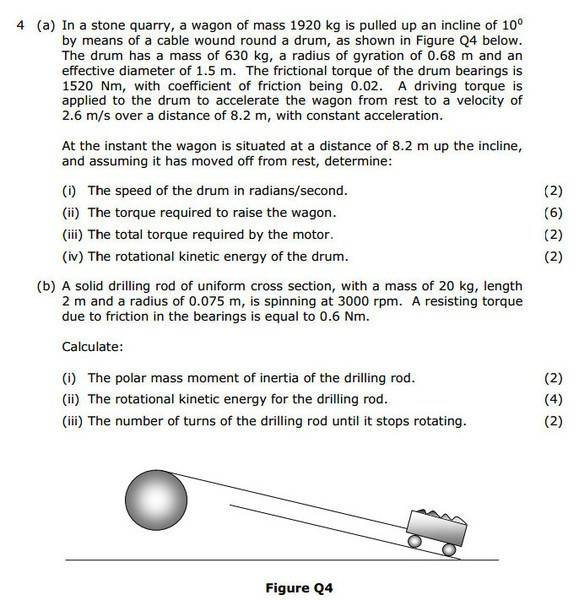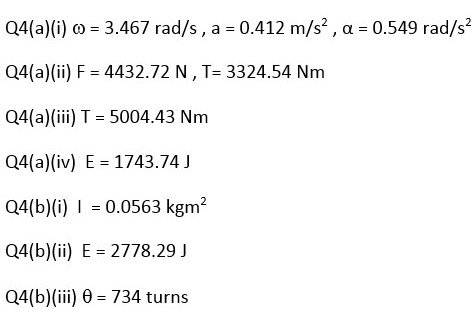# Torque, Energy, Number of Turns

• smr101
In summary, the conversation discusses the problem of calculating the force required for a wagon with a rotating drum attached to it. The solution suggests finding the torques acting on the drum and summing them instead of the forces. The equation for torque and angular acceleration is mentioned, and it is recommended to read up on moment of inertia to better understand the problem. Similarities between linear motion and rotation are also highlighted.

#### smr101

Hi, I'm having problems with 4. (a)(iii) and (b)(iii).

Question and solution attached below.

For 4.(a)(iii) this is what I've tried (for mass I've used the mass of the wagon plus drum):

F = Fu + Ff + Fw
= (ma) + (mg x cosangle x friction coefficient) + (mg x sinangle)
= 2550 x 0.412 + 2550 x 9.81 x cos10 x 0.02 + 2550 x 9.81 x sin10
= 1050.6 + 419.8 + 13608.96
= 15079.4Nm

(b)(iii)

Help is much appreciated.For 4.(a)(iii) this is what I've tried (for mass I've used the mass of the wagon plus drum):

That's not correct, you can't add the mass of the drum to the wagon. The drum isn't accelerating in a straight line, it's rotating.

First off don't sum the forces. Work out all the torques acting on the drum and sum them instead. For example one of the torques will be due to the tension in the cable. Another will be the torque required to accelerate the drum (angular acceleration). Find the equation that relates torque and angular acceleration.

Edit: There are other torques that have to be overcome. Make a list.

I see you have two questions/threads running. Looking at both of these threads it seems the difficulty you are having is the same in both cases. Perhaps read up on moment of inertia and understand the similarity between the equations for linear motion and rotation...

Linear.......>.......Rotation

Force = mass * acceleration.......Torque = moment of inertia * angular acceleration

Work = force * displacement.......Work = torque * angular displacement

Power = force * displacement/time......Power = torque * angular displacement/time
Power = force * velocity.........Power = torque * angular velocity